Special Offer - Enroll Now and Get 2 Course at ₹25000/- Only Explore Now!# Control Statements in Java

May 2nd, 2019

## Control Statements in Java

Usually, every program flow has top to bottom approach or vice-versa. But, if we can control the flow of a program, is by using Control statements. And the control statements include :

1. Decision making
2. Looping
3. Branching

### Decision Making:

Just like in our life when multiple options are available for a particular scenario/situation, we analyze and take right decisions and move forward based on that, in the same way in programming also there comes situations where we need to move the program forward based on the right decisions which is been taken among the multiple choices available.
The statements for decision making are:

• if
• if-else
• else-if ladder
• nested if
• switch case

#### Key points:

• The expressions used as conditions should return only boolean values.
• Relational and Logical operators are used to express conditions.
• If one statement is to be executed, that can be written without using curly braces {}, but if multiple statements has to be executed curly braces must be used.

#### if Statement:

if Statement is used to decide between two conditions in a way like, if the condition/conditions given in ‘if’ are true, executes statement 1 otherwise executes statements other than ‘if’.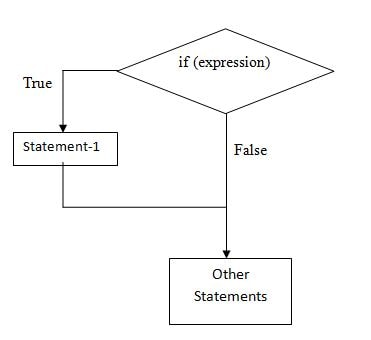##### Syntax:
 ```// if condition1= true; executes Statement-1 & other statements // if condition1=false; executes only other statements & skips the if block. if (condition1) { Statement-1; } other statements - -```

##### Example:

This is a program where if the user’s points in a competition are above 600, then the user will be displayed with a message “Congrats! You are selected for next level!” & with the message “Thank you for participating!” And if the user’s points are below 600, then only the message “Thank you for participating!” will be displayed.

 ```class Sample_If{ public static void main(String[] args) { int points=840; if (points>600) { System.out.println("Congrats! You are selected for next level!”); } System.out.println("Thank you for participating!"); } }```

#### if-else Statement:

if-else statement is used to take decisions between two conditions in a way like, if the expression is true execute Statement 1 or else executes Statement 2.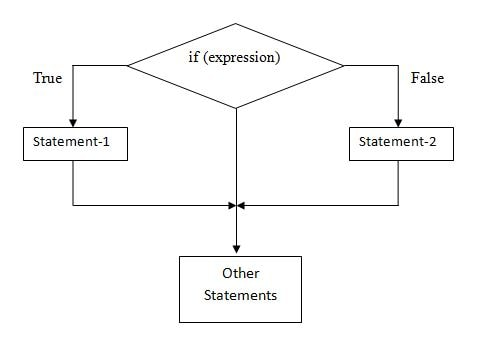##### Syntax:
 ```// if condition1= true; executes Statement-1 & other statements, // if condition1=false; executes Statement-2 & other statements. if (condition1) { Statement-1; } else { Statement-2; } other statements - -```
##### Example:

This is a program where if the user’s points in a competition are above 600, then the user will be displayed with a message “Congrats! You are selected for next level!” & with the message “Thank you for participating!” And if the user’s points are below 600, then the user will be displayed with a message “So sorry! Better luck next time!” & with the message “Thank you for participating!”

 ```class Sample_If { public static void main(String[] args) { int points=550; if (points>600) { System.out.println("Congrats! You are selected for next level!”); } else { System.out.println(“So sorry! Better luck next time!”); } System.out.println("Thank you for participating!"); } }```

#### else-if Ladder:

else-if Ladder is used to take decisions between multiple (more than two) conditions in a way like, if the first expression is true executes Statement 1 else checks for next expression to be true, if not then checks for the next & so on. If none are true, finally executes else part and other statements following that.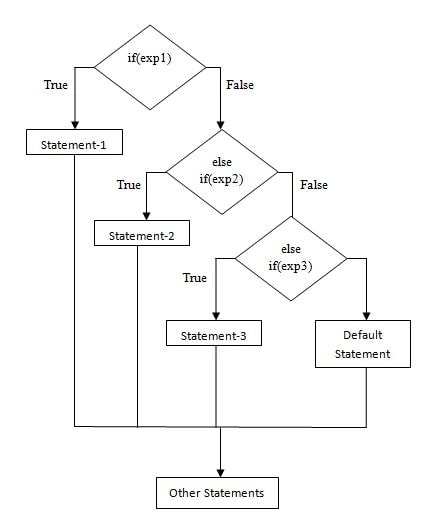##### Syntax:
 ```// if condition1= true; executes Statement-1 & other statements, // if condition2=true; executes Statement-2 & other statements, so on //if all condition are false, executes else part & other statements. if (condition1) { Statement-1; } else if(condition2) { Statement-2; } else if(condition3) .        (condition4) .        (condition5),etc else { Default statement; } other statements - - ```
##### Example:

This is a program which states different levels of marks category of students using else-if ladder. In this, the statement matching the given mark will be executed along with the statement “Wishing you for a best future!”.

 ```public class Marks { public static void main(String[] args) { int mark=95; if(mark>=75) System.out.println("Distinction"); else if(mark>=60) System.out.println("First Class"); else if(mark>=50) System.out.println("Pass"); else System.out.println("Fail"); System.out.println("Wishing you for a best future!"); } }```

#### Nested if Statement:

Nested if Statement is used to take decisions between multiple (more than two) conditions in a way like, if the first expression is true, within that ‘if’ block, its sub level if statements will be executed. If the first expression is false then the control goes to the else part which can also have many sublevel if ‘s. Therefore, whichever statement matches will be executed.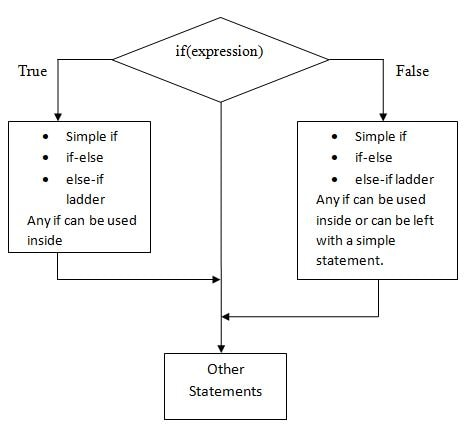##### Syntax:

// If condition1= true; executes the inner sub level if statements & if condition1=false; executes if any ‘if statements’ exists inside else part or else just prints the statement available there.

 ```if (condition1) { Simple if or if-else or else-if ladder, any if can be inside. } else { Simple if or if-else or else-if ladder, any if can be inside or can be left with a simple statement. } other statements - -```
##### Example:

Here the previous program is written using Nested-if statement. In this if condition-1 is true, then the sub level condition-2 is executed. If it is satisfied then condition-3 is executed. If any condition becomes false then its corresponding else part will be executed.

 ```public class Marks2 { public static void main(String[] args) { int mark=87; if(mark>50) // condition-1 { if(mark>60) // condition-2 { if (mark>75) // condition-3 { System.out.println("Distinction"); } else { System.out.println("First Class"); } } else { System.out.println("Pass"); } } else { System.out.println("Fail"); } } }```

#### Switch case Statement:

Switch Case is used to execute different parts of code based on the value of the expression.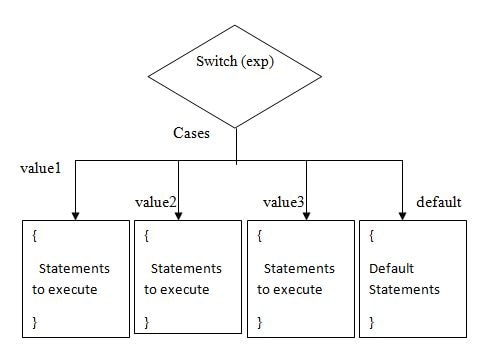##### Syntax:
 ```switch(expression) { case value1: { Set of statements break; } case value2: { Set of statements break; } case value3: { Set of statements break; } default: { Set of statements break; } }```

##### Example:

This is a program to display the weekdays to the user. In this, Monday is assigned to String variable day. In switch, day is given as an expression which contains the value Monday. If that value matches with any of the case values given below then that set of statements will be executed.

#### Break:

It is a branching statement, used to break the flow of execution then and there itself then jumps/exits out of that block. Used to abruptly stop the program flow and jumps out of the block.

 ```package website; public class SampleSwitch { public static void main(String[] args) { String day="Monday"; switch(day) { case "Monday": System.out.println("Today is Monday"); break; case "Tuesday": System.out.println("Today is Tuesday"); break; case "Wednesday": System.out.println("Today is Wednesday"); break; case "Thursday": System.out.println("Today is Thursday"); break; case "Friday": System.out.println("Today is Friday"); break; case "Saturday": System.out.println("Today is Saturday"); break; default: System.out.println("Today is Sunday which is a Holiday!"); break; } } }```

### Looping:

It is the process of executing the same set of statements repeatedly until the desired number of times or based on a given boolean condition. This process is also called as Iteration.

• for
• while
• do-while

#### for loop :

In this a value is initialized, then each time while repeating the set of statements it is incremented/decremented, then the statements are executed repeatedly until the given condition becomes false.

##### Syntax:
 ```for(initialization; condition/expression; increment/decrement) { Set of statements }```
##### Example:

Here, we are printing numbers from 1 to 10. First i=0 then assigned to 1. Condition checks that i>0 and i<=10, then only the next set of statements executes, using for loop.

 ```public class Loop { public static void main(String[] args) { int i=0; for(i=1;(i>0)&&(i<=10);i++) { System.out.print("\t"+i); } } }```

#### while loop:

It is same as for loop, but here the difference is we use ‘while’ keyword & syntax differs.

#### Syntax:

 ```initialization while(condition/expression) { Set of statements increment/decrement }```
##### Example:

Here, we are printing numbers from 1 to 10. First i=0 then assigned to 1. Condition checks that i>0 and i<=10, then only the next set of statements executes, using while loop.

```public class Loop
{
public static void main(String[] args)
{
int i=0;
i=1;
while((i>0)&&(i<=10))
{
System.out.print("\t"+i);
i++;
}
}
}```

### do-while:

This is also same as while loop but the only difference is the set of statements are executed once without checking the condition in while and condition is checked at last, not initially.

#### Syntax:

 ```initialization do { Set of statements increment/decrement } while(condition/expression)```
##### Example:

Here, we are printing numbers from 1 to 10. First i=0 then assigned to 1. Condition checks that i>0 and i<=10, then only the next set of statements executes, using do-while loop.

 ```public class Loop { public static void main(String[] args) { int i=0; i=1; do { System.out.print("\t"+i); i++; }while((i>0)&&(i<=10)); } }```

#### Difference between while and do-while loop:

Using While: Here, the output will be empty.

 ```public class Loop { public static void main(String[] args) { int i=0; // i=1; while((i>0)&&(i<=10)) { System.out.print("\t"+i); i++; } } }```
Using do-while: Here, the output will be : 0    1          2          3          4          5          6          7          8     9   10
 ```public class Loop { public static void main(String[] args) { int i=0; //i=1; do { System.out.print("\t"+i); i++; }while((i>0)&&(i<=10)); } }        ```

### Branching:

Branching statements are used to jump from the current executing loop.
The statements for branching are:

• break : It is a branching statement, used to break the flow of execution then and there itself then jumps/exits out of that block. Used to abruptly stop the program flow and jumps out of the block.
• continue : It is a branching statement, used to continue the execution of loop.
• return : It is a branching statement, returns the value to the method call.
Social Share: